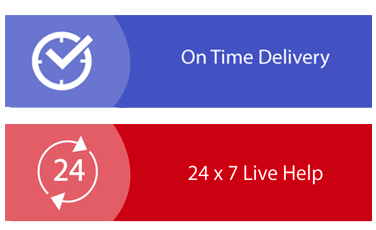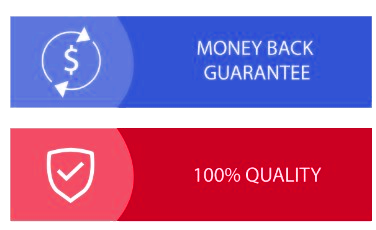× Limited Time Offer ! FLAT 30-40% off - Grab Deal Before It’s Gone. Order Now

## Qustion

### Learning Activity 3.1: Loops

There are a number of activities in this module and you are expected to complete all of these in the labs or in class or in your own time. The first of these is to reproduce any and all of the worked examples in the module content and to review the readings and videos. Then, work your way through these exercises:

1. Write a program to prompt for and read a sequence of non-negative numbers (with a negative number to end input).

Example:

Enter a number (negative to quit): 10.0 Enter a number (negative to quit): 7.5 Enter a number (negative to quit): 3.5 Enter a number (negative to quit): -1

2. Write a program to prompt for and read a sequence of non-negative numbers (with a negative number to end input) and then print the least non- negative input number. If the first number read is negative, print "No numbers.". Round the least number off to one decimal place.
Example:

Enter a number (negative to quit): 10.0

Enter a number (negative to quit): 7.5 Enter a number (negative to quit): 3.5 Enter a number (negative to quit): -1 Least number is: 3.5

3. Write a guessing game where the user has to guess a secret number. After every guess, the program tells the user whether his number was too large or too small. At the end the number of tries needed should be printed. You may count only as one try if the user inputs the same number consecutively. Note that for this question, you need to use a combination of loops and conditional statements.

### Learning Activity 3.2: Loops advanced

There are a number of activities in this module and you are expected to complete all of these in the labs or in class or in your own time. The first of these is to reproduce any and all of the worked examples in the module content and to review the readings and videos. Then, work your way through these exercises:

1. Write a program that asks the user for a counter upper bound, and prints the odd numbers between 1 and the user inserted value.

2. Given an asset original price, the percentage with which the asset depreciates every year and the number of years elapsed, write an algorithm that calculates the actual value of the asset at the moment.

Assume that the depreciation percentage is fixed but each year it is calculated on the current actual value of the asset.

3. Given an asset original price, the percentage with which the asset depreciates every year and the actual value of the asset, write an algorithm that calculates the number of years elapsed for the asset to reach this value. Assume that the depreciation percentage is fixed but each year it is calculated on the current actual value of the asset.

4. Write an algorithm that helps investors estimate their bank account balance after a certain number of years. The program should ask the investor to insert the original account balance and the number of years after which they would like to estimate their balance. Note that a Sydney banks offer a fixed yearly interest of 2%.

5. Write an algorithm that helps a frequent traveller employee redeem his expenses. The program should ask the employee about the number of days he has travelled. The program will then repeatedly ask the employee to insert his daily expenses for each day he travelled independently. The program should print out to total sum of the expenses.

## Solution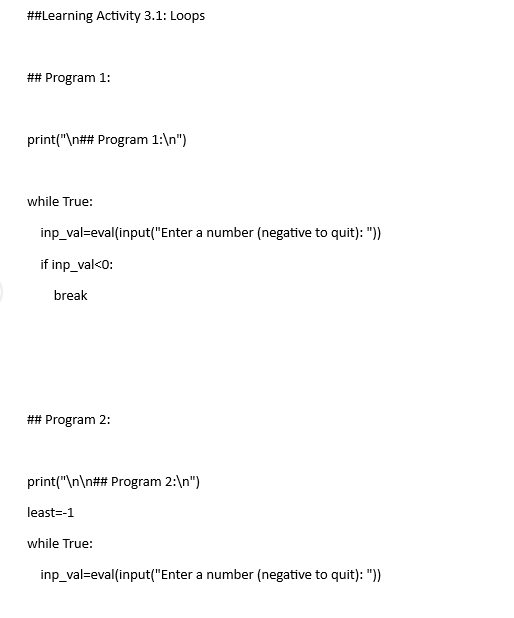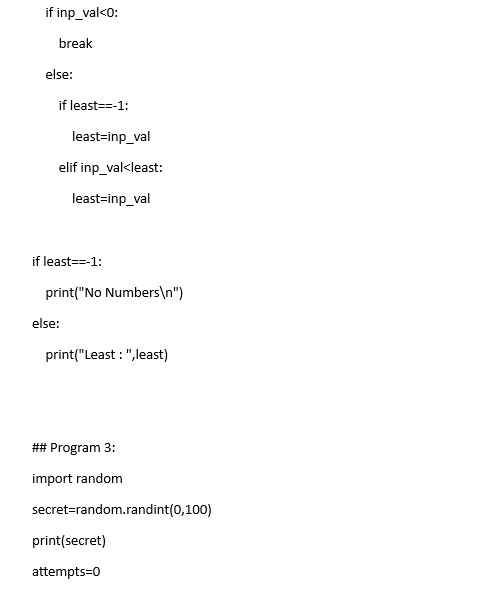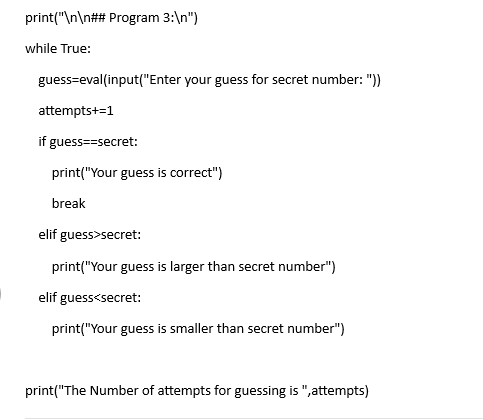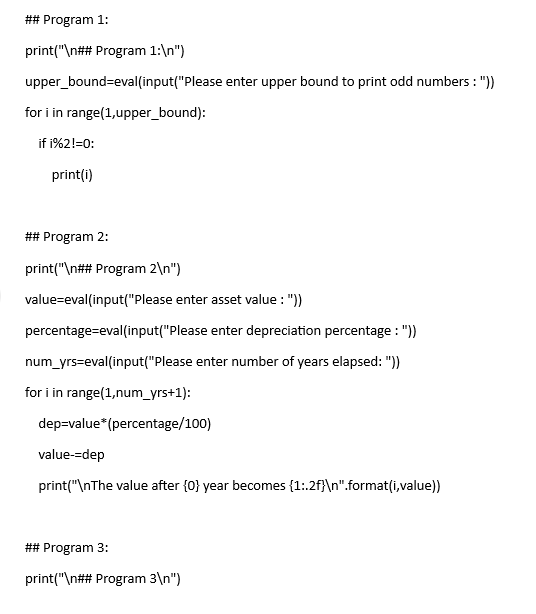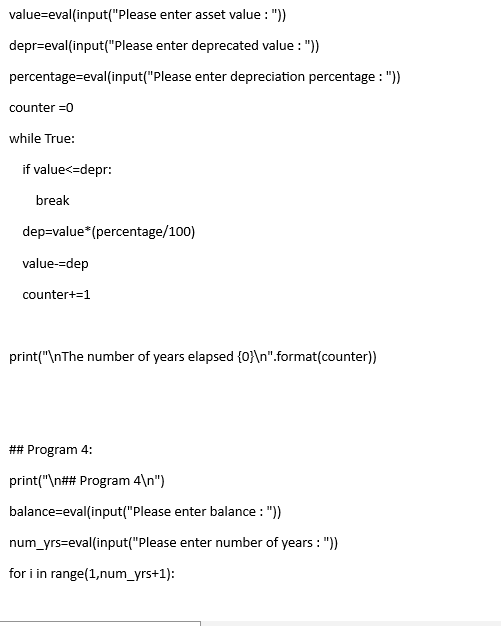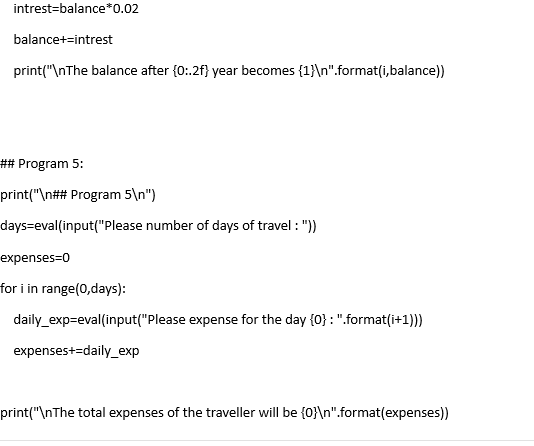Fill the form to continue reading